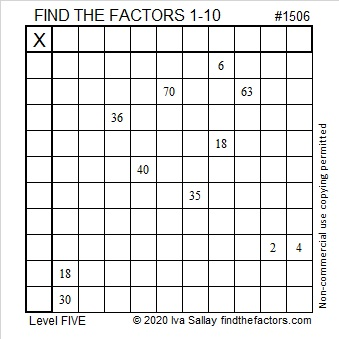# 1506 and Level 5

Contents

### Today’s Puzzle:

Can you find the factors 1 to 10 in a logical order so that the given clues are the products of those factors? Don’t let any of the clues trick you!### Factors of 1506:

• 1506 is a composite number.
• Prime factorization: 1506 = 2 × 3 × 251.
• 1506 has no exponents greater than 1 in its prime factorization, so √1506 cannot be simplified.
• The exponents in the prime factorization are 1, 1, and 1. Adding one to each exponent and multiplying we get (1 + 1)(1 + 1)(1 + 1) = 2 × 2 × 2 = 8. Therefore 1506 has exactly 8 factors.
• The factors of 1506 are outlined with their factor pair partners in the graphic below.### Pythagorean triples with 1506:

1506 is not the sum or the difference of two squares but it is still part of two Pythagorean triples:
1506-567008-567010, calculated from 2(753)(1), 753² – 1², 753² + 1², and
1506-62992-63010, calculated from 2(251)(3), 251² – 1², 251² + 1².

This site uses Akismet to reduce spam. Learn how your comment data is processed.